# The Chemistry of Acids and BasesPage 4

#### WATCH ALL SLIDES

pH = - log (1.0 x 10-11) = 11.00

Slide 34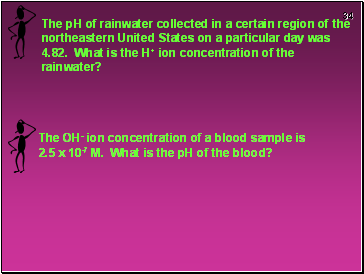The pH of rainwater collected in a certain region of the northeastern United States on a particular day was 4.82. What is the H+ ion concentration of the rainwater?

Slide 35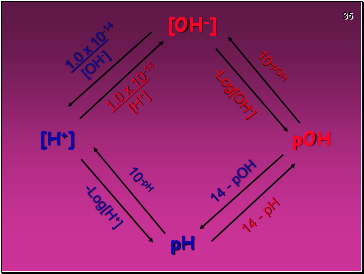[OH-]

[H+]

pOH

pH

10-pOH

10-pH

-Log[H+]

-Log[OH-]

14 - pOH

14 - pH

1.0 x 10-14

[OH-]

1.0 x 10-14

[H+]

Slide 36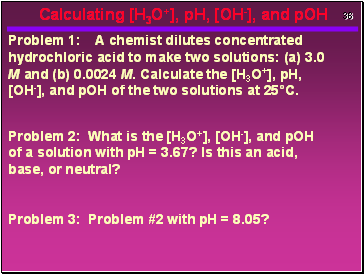Calculating [H3O+], pH, [OH-], and pOH

Problem 1: A chemist dilutes concentrated hydrochloric acid to make two solutions: (a) 3.0 M and (b) 0.0024 M. Calculate the [H3O+], pH, [OH-], and pOH of the two solutions at 25°C.

Problem 2: What is the [H3O+], [OH-], and pOH of a solution with pH = 3.67? Is this an acid, base, or neutral?

Problem 3: Problem #2 with pH = 8.05?

Slide 37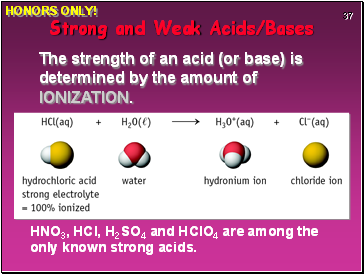HNO3, HCl, H2SO4 and HClO4 are among the only known strong acids.

Strong and Weak Acids/Bases

The strength of an acid (or base) is determined by the amount of IONIZATION.

HONORS ONLY!

Slide 38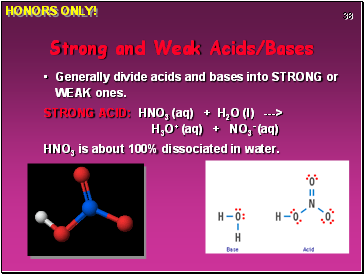Strong and Weak Acids/Bases

Generally divide acids and bases into STRONG or WEAK ones.

STRONG ACID: HNO3 (aq) + H2O (l) ---> H3O+ (aq) + NO3- (aq)

HNO3 is about 100% dissociated in water.

HONORS ONLY!

Slide 39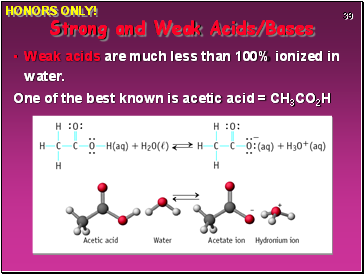Weak acids are much less than 100% ionized in water.

One of the best known is acetic acid = CH3CO2H

Strong and Weak Acids/Bases

HONORS ONLY!

Slide 40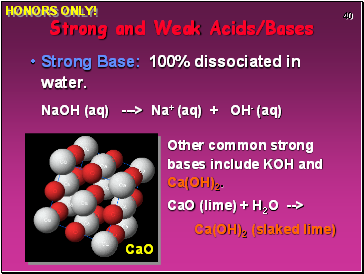Strong Base: 100% dissociated in water.

NaOH (aq) ---> Na+ (aq) + OH- (aq)

Strong and Weak Acids/Bases

Other common strong bases include KOH and Ca(OH)2.

CaO (lime) + H2O -->

Ca(OH)2 (slaked lime)

HONORS ONLY!

Slide 41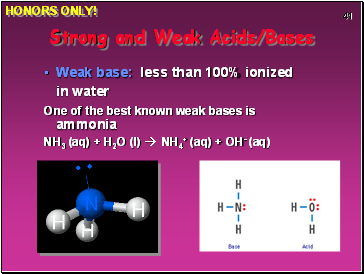Weak base: less than 100% ionized in water

One of the best known weak bases is ammonia

NH3 (aq) + H2O (l)  NH4+ (aq) + OH- (aq)

Strong and Weak Acids/Bases

HONORS ONLY!

Slide 42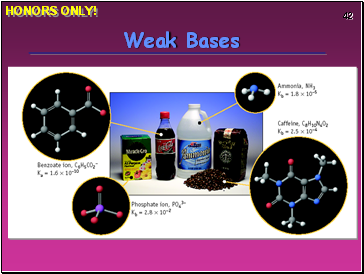Weak Bases

HONORS ONLY!

Slide 43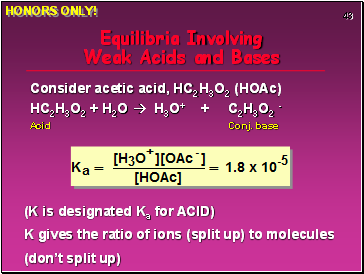Equilibria Involving Weak Acids and Bases

Consider acetic acid, HC2H3O2 (HOAc)

HC2H3O2 + H2O  H3O+ + C2H3O2 -

Acid Conj. base

(K is designated Ka for ACID)

Go to page:
1  2  3  4  5  6  7  8